Data Structures and Algorithms with Object-Oriented Design Patterns in C### Big Oh Fallacies and Pitfalls

Unfortunately, the way we write big oh notation can be misleading to the naıve reader. This section presents two fallacies which arise because of a misinterpretation of the notation.

Fallacy  Given that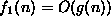and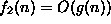, then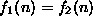.

Consider the equations: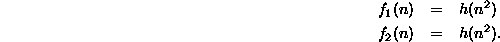Clearly, it is reasonable to conclude that.

However, consider these equations: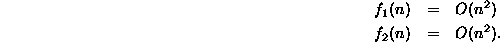It does not follow that. For example,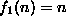and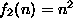are both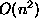, but they are not equal.

Fallacy  If f(n)=O(g(n)), then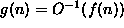.

Consider functions f, g, and h, such that f(n)=h(g(n)). It is reasonable to conclude that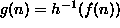provided that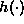is an invertible function. However, while we may write f(n)=O(h(n)), the equationis nonsensical and meaningless. Big oh is not a mathematical function, so it has no inverse!

The reason for these difficulties is that we should read the notation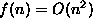as ``f(n) is big oh n squared'' not ``f(n) equals big oh of n squared.'' The equal sign in the expression does not really denote mathematical equality! And the use of the functional form,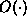, does not really mean that O is a mathematical function!Copyright © 2001 by Bruno R. Preiss, P.Eng. All rights reserved.